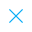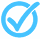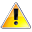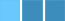Call Support +91-85588-96644

Keep me logged in
You can't leave Captcha Code empty
By submitting this form, you agree to the Terms & Privacy Policy.
ORTests given

OR# Theory of Equations (Practice Exercise - 4)

Questions

Time

Highest
score

Level

## ENGLISH

Language

Topics Covered:Quadratic EquationsQuadratic EquationsQuadratic EquationsQuadratic EquationsQuadratic EquationsQuadratic EquationsQuadratic EquationsQuadratic EquationsQuadratic EquationsQuadratic EquationsThis is a concept-building practice test and may not have exact structure as you would expect in the actual exam. Please exercise your discretion to attempt it or go to structured Featured Section.

Description:
Theory of Equations -Practice Exercise - 4